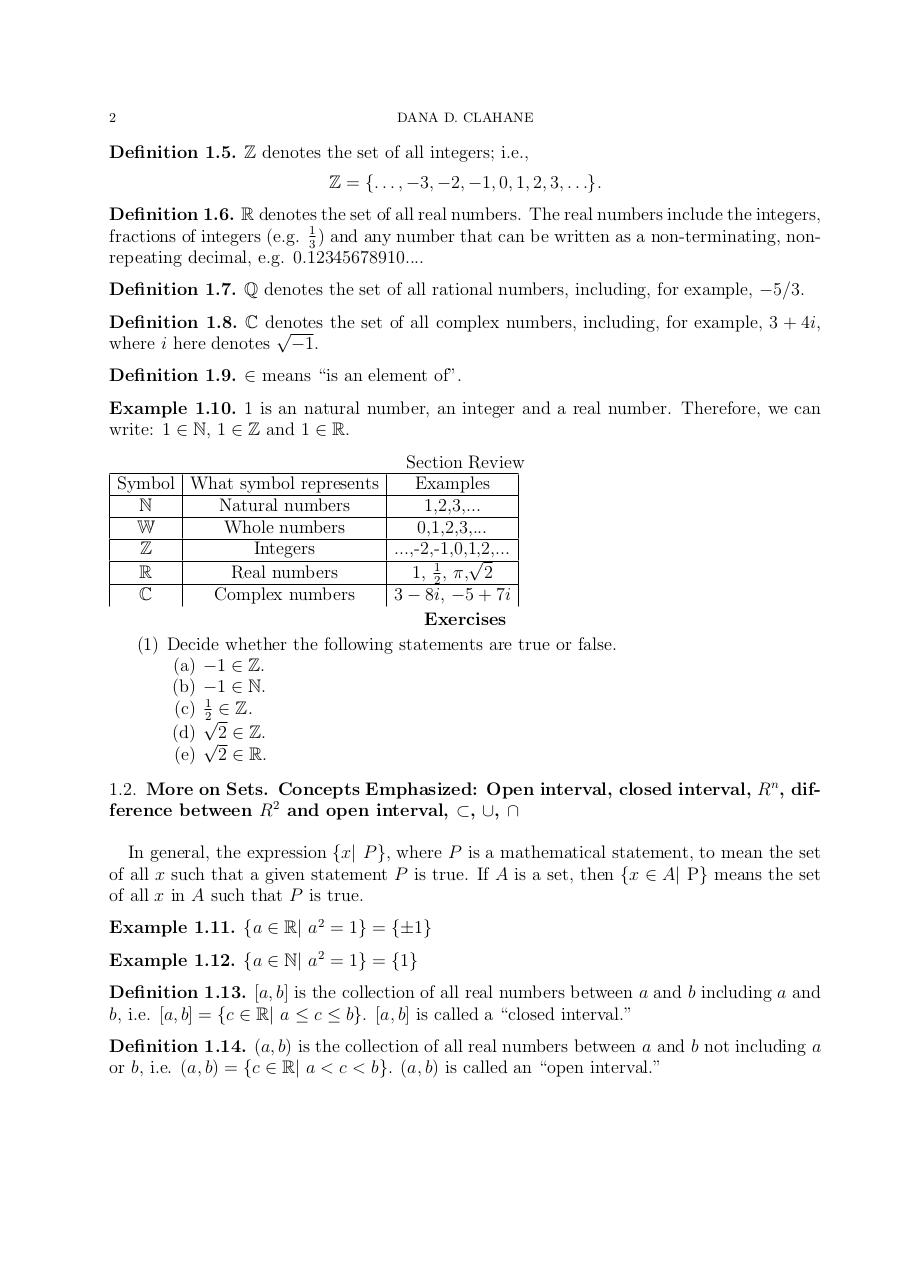# ODELAClahane061416.pdfPage 1 23470

#### Text preview

2

DANA D. CLAHANE

Definition 1.5. Z denotes the set of all integers; i.e.,
Z = {. . . , −3, −2, −1, 0, 1, 2, 3, . . .}.
Definition 1.6. R denotes the set of all real numbers. The real numbers include the integers,
fractions of integers (e.g. 13 ) and any number that can be written as a non-terminating, nonrepeating decimal, e.g. 0.12345678910....
Definition 1.7. Q denotes the set of all rational numbers, including, for example, −5/3.
Definition 1.8. C denotes
the set of all complex numbers, including, for example, 3 + 4i,

where i here denotes −1.
Definition 1.9. ∈ means “is an element of”.
Example 1.10. 1 is an natural number, an integer and a real number. Therefore, we can
write: 1 ∈ N, 1 ∈ Z and 1 ∈ R.
Section Review
Symbol What symbol represents
Examples
N
Natural numbers
1,2,3,...
W
Whole numbers
0,1,2,3,...
Z
Integers
...,-2,-1,0,1,2,...

R
Real numbers
1, 12 , π, 2
C
Complex numbers
3 − 8i, −5 + 7i
Exercises
(1) Decide whether the following statements are true or false.
(a) −1 ∈ Z.
(b) −1 ∈ N.
(c) 12 ∈ Z.

(d) √2 ∈ Z.
(e) 2 ∈ R.
1.2. More on Sets. Concepts Emphasized: Open interval, closed interval, Rn , difference between R2 and open interval, ⊂, ∪, ∩
In general, the expression {x| P }, where P is a mathematical statement, to mean the set
of all x such that a given statement P is true. If A is a set, then {x ∈ A| P} means the set
of all x in A such that P is true.
Example 1.11. {a ∈ R| a2 = 1} = {±1}
Example 1.12. {a ∈ N| a2 = 1} = {1}
Definition 1.13. [a, b] is the collection of all real numbers between a and b including a and
b, i.e. [a, b] = {c ∈ R| a ≤ c ≤ b}. [a, b] is called a “closed interval.”
Definition 1.14. (a, b) is the collection of all real numbers between a and b not including a
or b, i.e. (a, b) = {c ∈ R| a &lt; c &lt; b}. (a, b) is called an “open interval.”